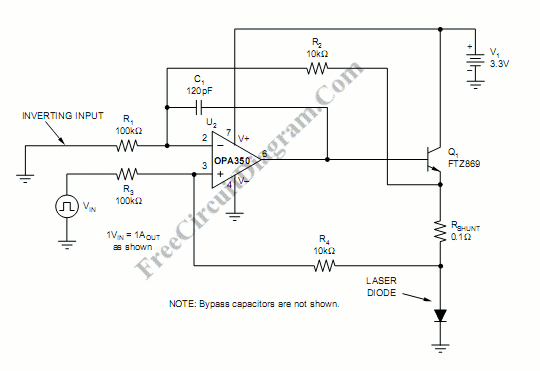# Laser Diode DriverA  laser diode driver can be implemented using voltage-controlled current source.  This simple  linear laser diode driver gives cleaner drive current than a switched (PWM) drivers. Here is the schematic diagram of the circuit:A Howland current pump with a current booster is used as the basic of this laser diode driver. Q1 is used as the current booster on the output of a R-R CMOS OPA350 op amp (U2). Voltage drop across a shunt resistor (RSHUNT) in series with the laser diode is measured differentially to sense the current. An analog voltage from a potentiometer of from a microprocessor controlled DAC can be fed to VIN to control the laser diode current.  Using the component values as shown, the scale factor is 1V input equals 1A output. Other values of scale factor (VIN/IOUT) can be set by choosing different resistor values using the equation:

VIN/IOUT=RSHUNT(R3/R4), with R1=R3, and R2=R4.

[Circuit schematic diagram source: Burr-Brown Products Application Report]